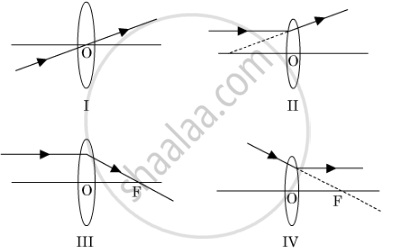Share

# A student has obtained a magnified image of a flame on a screen using a convex lens. To draw the corresponding ray diagram to show the image formation, which of the following two rays whose paths after refraction are shown, should h - CBSE Class 10 - Science

ConceptRefraction of Light Image Formation in Lenses Using Ray Diagrams - Convex Lens

#### Question

A student has obtained a magnified image of a flame on a screen using a convex lens. To draw the corresponding ray diagram to show the image formation, which of the following two rays whose paths after refraction are shown, should he select ?(A) I and II

(B) II and III

(C) III and IV

(D) I and III

#### Solution

A convex lens converges the refracting ray. Therefore, rays I and III represent the path of the refracting ray from a convex lens(converging lens). As rays II and IV show the refracting ray being diverged, the ray diagrams are incorrect.

Hence, the correct option is D.

Is there an error in this question or solution?

#### APPEARS IN

Solution A student has obtained a magnified image of a flame on a screen using a convex lens. To draw the corresponding ray diagram to show the image formation, which of the following two rays whose paths after refraction are shown, should h Concept: Refraction of Light - Image Formation in Lenses Using Ray Diagrams - Convex Lens.
S